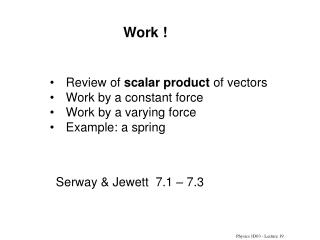DownloadDownload PresentationWork !

# Work !

Télécharger la présentation## Work !

- - - - - - - - - - - - - - - - - - - - - - - - - - - E N D - - - - - - - - - - - - - - - - - - - - - - - - - - -
##### Presentation Transcript

1. Work ! • Review of scalar product of vectors • Work by a constant force • Work by a varying force • Example: a spring Serway & Jewett 7.1 – 7.3 Physics 1D03 - Lecture 19

2. Work and Energy Newton’s approach: - acceleration at any instant is caused by forces Energy approach: Net work = increase in kinetic energy • equivalent to Newton’s dynamics • scalars, not vectors • compares energies “before and after” Physics 1D03 - Lecture 19

3. Math Review The scalar product or dot product of two vectors gives a scalar result: vector • vector = scalar  Physics 1D03 - Lecture 19

4. and ScaIar product and cartesian components: (note the right-hand-side is a single scalar) To prove this, expand using the laws of arithmetic (distributive, commutative), and notice that since, i, j, k are mutually perpendicular since they are unit vectors Physics 1D03 - Lecture 19

5. A constant force is applied to an object while it undergoes a displacement The work done by is : MATH QUIZ Physics 1D03 - Lecture 19

6. Work   Work by a constant force F during a displacement s: Work = (component of F parallel to motion) x (distance) We can also write this as: Work = F• s = Fscos(θ) Units : N • m = joule (J) This is the “scalar product”, or “dot product”. Work is a scalar.If work is done on a system, W is positive (eg: lifting an object).If work is done by a system, W is negative (eg: object falling) Physics 1D03 - Lecture 19

7. Example (massless pulleys, no friction) FP s = 2 m 100 N How much work is done on the rope by Fp? How much work done by the upward force on the ball? Physics 1D03 - Lecture 19

8. a) 20 J b) c) 40 J Quiz Fg = 5 N P = 10 N 2 m 3 m The two forces, P and Fg are constant as the block moves up the ramp. The total work done by these two forces combined is: Physics 1D03 - Lecture 19

9. Quiz 2.5 m Fp = 120 w= 100 N 5 3 fk = 50 N 4 n The block is dragged 2.5 m along the slope. Which forces do positive work? negative work? zero work? Physics 1D03 - Lecture 19

10. Quiz 2.5 m Fp = 120 w= 100 N 5 3 fk = 50 N 4 n The block is dragged 2.5 m along the slope. Find : • work done by Fp • work done by fk • work done by gravity • work done by normal force • Total work on the block Physics 1D03 - Lecture 19

11. Forces which are not constant: Example: How much work is done to stretch a spring scale from zero to the 20-N mark (a distance of 10 cm)? We can’t just multiply “force times distance” because the force changes during the motion. Our definition of “work” is not complete. Varying force: split displacement into short segments over which F is nearly constant. F(x) x For each small displacement Dx, the work done is approximately F(x) Dx, which is the area of the rectangle. F D x Physics 1D03 - Lecture 19

12. Work is the area (A) under a graph of force vs. distance We get the total work by adding up the work done in all the small steps. As we let Dx become small, this becomes the area under the curve, and the sum becomes an integral. F(x) F(x) A x x Split displacement into short steps Dx over which F is nearly constant... Take the limit as Dx 0 and the number of steps   Physics 1D03 - Lecture 19

13. In 1D (motion along the x-axis): Another way to look at it: Suppose W(x) is the total work done in moving a particle to position x. The extra work to move it an additional small distance Dx is, approximately, DW  F(x) Dx. Rearrange to get In the limit as Dx goes to zero, Physics 1D03 - Lecture 19

14. Example: An Ideal Spring. Hooke’s Law: The tension in a spring is proportional to the distance stretched. or, |F| = k|s| The spring constant k has units of N/m Directions: The force exerted by the spring when it is stretched in the +x direction is opposite the direction of the stretch (it is a restoring force): F = -kx Physics 1D03 - Lecture 19

15. Example: Work by a Spring Fs Fs= - kx Find a function W(x) so that Physics 1D03 - Lecture 19

16. Quiz A physicist uses a spring cannon to shoot a ball at a stuffed gorilla. The cannon is loaded by compressing the spring 20 cm. The first 10 cm of compression requires work W. The work required for the next 10 cm (to increase the compression from 10 cm to 20 cm) would be • W • 2W • 3W • 4W Physics 1D03 - Lecture 19

17. Summary For a constant force, Work = F• s = (component of force parallel to motion)  (distance) For a non-constant force (1-D) : Work = Fx(x) dx Practice problems: Chapter 7, Problems 1, 7, 11, 13(5th ed) Problems 5, 11, 15, 17 Read section 7.2 (scalar product). Physics 1D03 - Lecture 19